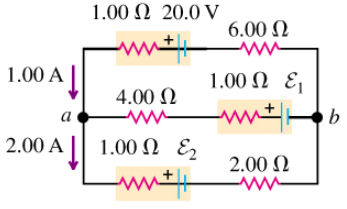# Problem: (a) Find the emf ε1 in the circuit of the figure (Figure 1) .(b) Find the emf ε2 in the circuit of the figure.(c) Find the potential difference of point b relative to point a

###### FREE Expert Solution

We need three equations—Kirchhoff's loop rule:

$\overline{){\mathbf{\sum }}{\mathbit{V}}{\mathbf{=}}{\mathbf{0}}}$ around each loop

Kirchhoff's junction rule:

$\overline{){\mathbf{\sum }}{{\mathbit{i}}}_{\mathbit{i}\mathbit{n}}{\mathbf{=}}{\mathbf{\sum }}{{\mathbit{i}}}_{\mathbit{o}\mathbit{u}\mathbit{t}}}$ at each junction

and Ohm's Law

$\overline{){\mathbit{V}}{\mathbf{=}}{\mathbit{i}}{\mathbit{R}}}$

We don't know i3, but we know the other two currents, all the resistances, and one of three EMFs/voltages. Three unknowns: we need three equations.

90% (84 ratings)###### Problem Details(a) Find the emf ε1 in the circuit of the figure (Figure 1) .

(b) Find the emf ε2 in the circuit of the figure.

(c) Find the potential difference of point b relative to point a